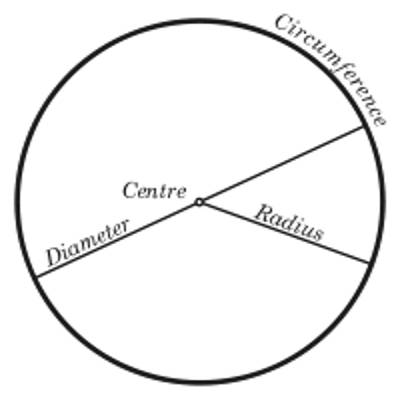A circle is a line forming a closed loop, every point on which is a fixed distance from a center point. Center is the point inside the circle. All points on the circle are equidistant (same distance) from the center point. The radius is the distance from the center to any point on the circle. It is half the diameter.

Important Properties on Circle

The two important properties of the circle are stated below:

1. The ratio of the circumference to the diameter of any circle is constant and the value of this constant is denoted by the Greek letter π.

Therefore, the circumference of any circle/diameter of that circle = constant = π

or, the circumference of any circle = π × diameter of that circle.

If r be the radius of the circle then its diameter is 2r.

Therefore, the circumference of the circle = π ∙ 2r = 2πr.

The constant quantity π is an incommensurable number i.e., it cannot be expressed as the ratio of two positive integers. An approximate value or π is 27/7; a more accurate value of π is 355/133 or, 3.14159 (correct to five places of decimals).1. Angles at the center of a circle are proportional to the lengths of the arcs which subtend those angles.

The above two important properties on the circle will help us to prove that a radian is a constant angle.

In geometry, a large number of facts about circles and their relations to straight lines, angles, and polygons can be proved. These facts are called the properties of the circle.

• Circles having equal radii are congruent.
• Circles having different radii are similar.
• The radius perpendicular to a chord bisects the chord.
• The chords equidistant from the center are equal in length.
• A tangent to a circle is at a right angle to the radius at the point of contact.
• Two tangents drawn on a circle from a point outside are equal in length.
• The angle subtended at the center of a circle by its circumference is equal to four right angles.
• Arcs of the same circle are proportional to their corresponding angles.
• Radii of the same circle or equal circles are equal.
• Equal chords have equal circumferences.
• The diameter of a circle is the longest chord.

Information Source: